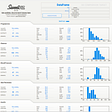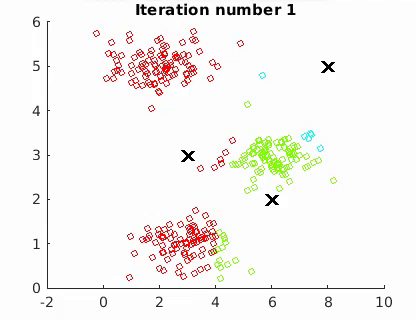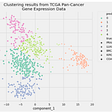# 2-D Visualization using Principal Component Analysis (PCA) on MNIST dataset

Going to perform this using 2 methods

Method 1 :We are going to perform it manually by computing eigen values and eigen vectors explicitly
Method 2 : PCA Using Sklearn.

# Method 1 : Compute Manually

## Step 1: import libraries , load dataset:

`import numpy as npimport pandas as pdimport matplotlib.pyplot as pltdf = pd.read_csv("mnist_train.csv")print("Shape of df:",df.shape)  # i.e. we have 60K data points and 784 features with [28*28] `

Shape of df: (60000, 785)

Separate Dependent and Independent features

`labels = df['label']data = df.drop("label",axis = 'columns')print("labels.shape:",labels.shape)#Dependent features with class labels from (0–9)print("data.shape:",data.shape)# Independent features`

labels.shape: (60000,)
data.shape: (60000, 784)

## Step 2 : Data Preprocessing using sklearn.preprocessing

As soon as we got our data we do column Standardization using sklearn.preprocessing

Q] What is Column standardization ?

>>let say we have fj feature with [x1 to xn] values accordingly. we will transform it to [x1` to xn`] features such that where mean of each xi` = 0 and std-dev of each xi`= 1

Q2] How to compute col- standardization manually?

>>> lets , [xi` = xi -x_mean / x_std]

`from sklearn.preprocessing import StandardScalerstandardized_data = StandardScaler().fit_tranform(data)print(standardized_data.shape)`

(60000, 784)

So, till here we have standardized our data where each datapoint will have mean = 0 and stand-dev = 1

## Step 3 : Find Covariance Matrix i.e. S = X^T * X , where now S = [d x d] matrix with X^T =[d x n] , X = [n x d]

`sample_data = standardized_data# matrix multiplication using Numpycov_matrix = np.matmul(sample_data.T,sample_data)print("The shape of variance matrix = ", cov_matrix.shape)`

The shape of co- variance matrix = (784, 784)

## Step 4 : After we have done basic steps now its tike to compute top 2 Eigen values and Eigen Vectors as we are transforming data to 2-D.

Note: as we will use sklearn.linalg for computing eigen values and vectors where (eigh) func akways gives us eigen values and vectors in Ascending order i.e. from lower to higher.

`from scipy.linalg import eighvalue,vectors = eigh(cov_matrix ,eigvals=(782,783)) # top 2 in DESC ORDERprint(vectors.shape)  # (784 , 2) i.e. compute top 2 eigen valuesvectors = vectors.T     # tranforming vectors from (784, 2) to (2,784)print("Updated shape of eigen vectors = ",vectors.shape)`

(784, 2)

`# projecting the original data sample on the plane #formed by two principal eigen vectors by vector-vector multiplication.new_coordinates = np.matmul(vectors.T,sample_data.T)print("new_cordinates:", vectors.T.shape, " X ", sample_data.T.shape, " = ",new_coordinates.shape)`

new_cordinates: (2, 784) X (784, 60000) = (2, 60000)

`import pandas as pd# appending label to the 2d projected datanew_coordinates = np.vstack((new_coordinates, labels)).T# vstack = vertical stack# creating a new data frame for ploting the labeled points.dataframe = pd.DataFrame(data=new_coordinates, columns=("1st_principal", "2nd_principal", "label"))print(dataframe.head())`
`# ploting the 2d data points with seabornimport seaborn as snsn.FacetGrid(dataframe, hue="label", size=6).map(plt.scatter, '1st_principal', '2nd_principal').add_legend()plt.show()`

Observations : So, we have plotted Scatter-Plot with 1st_principal on X-axis & 2nd_principal on y-axis. here PCA gives not that well visualization but it tries it best by separating Class Label [9, 7,0,8], while other labels are not that well separated.

# Method 2 : PCA using Scikit-Learn

## Step 1 : from sklearn import decomposition

`# initializing the pcafrom sklearn import decompositionpca = decomposition.PCA()`

## Step 2 : Select number of pca components you need for Visualization

`pca.n_components = 2# select 2 components for 2-D visualization pca_data = pca.fit_transform(sample_data)# pca_reduced will contain the 2-d projects of simple datadataprint("shape of pca_reduced.shape = ", pca_data.shape)`

shape of pca_reduced.shape = (60000, 2)

# Step 3 : Visualization

`pca_data = np.vstack((pca_data.T, labels)).Tpca_df = pd.DataFrame(data=pca_data, columns=("1st_principal", "2nd_principal", "label"))sn.FacetGrid(pca_df, hue="label", size=6).map(plt.scatter, '1st_principal', '2nd_principal').add_legend()plt.show()`

Observation: It works exaclty same as what we have done manually above instead of that it just rotates the plot silghtly but as we discussed PCA tries to do its best by seperating some class labels but not all .

## More from Nihar Jamdar

Data Science Enthusiast

Love podcasts or audiobooks? Learn on the go with our new app.

## Analyzing Box Office Data## EDA in a single line of CODE## Exploring Trends in Substance Use through Data Visualization## Excel for Data Analytics-Is it still Relevant?## Betting tips with a new design## EURCAD Incomplete Bearish Sequence Pointing Lower## Implementation Of KNN In Machine Learning with Scikit-learn## Building a Fast Web Interface in Django for Data Entry## Nihar Jamdar

Data Science Enthusiast

## A33: Handling imbalanced classes in the dataset.## Image Matching with Shopee## K-Means Clustering in Python## Practical Guide To K-Means Clustering in Python